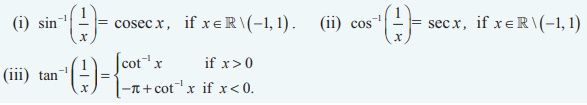Home | | Maths 12th Std | Summary

# Summary

Properties of Inverse Trigonometric Functions

SUMMARY

Inverse Trigonometric FunctionsProperties of Inverse Trigonometric Functions

Property-IProperty-IIProperty-III (Reciprocal inverse identities)Property-IV(Reflection identities)

(i) sin-1 (-x) = - sin-1 x ,if x [-1, 1] .

(ii)  tan-1 (-x) = - tan-1 x ,if x R.

(iii) cosec-1 (-x) = - cosec-1 x ,if |x| ≥ 1 or x  R \ (-1, 1) .

(iv) cos-1 (-x) = π - cos-1 x ,if x   [-1, 1] .

(v) sec-1 (-x) = π - sec-1 x ,if |x| ≥ 1 or x ∈ R \ (-1, 1) .

(vi) cot-1(-x) = π - cot-1 x , if x  R .

Property-V ( Cofunction inverse identities )Property-VIProperty-VIIProperty-VIIIProperty-IXProperty-XTags : Inverse Trigonometric Functions , 12th Mathematics : UNIT 4 : Inverse Trigonometric Functions
Study Material, Lecturing Notes, Assignment, Reference, Wiki description explanation, brief detail
12th Mathematics : UNIT 4 : Inverse Trigonometric Functions : Summary | Inverse Trigonometric Functions